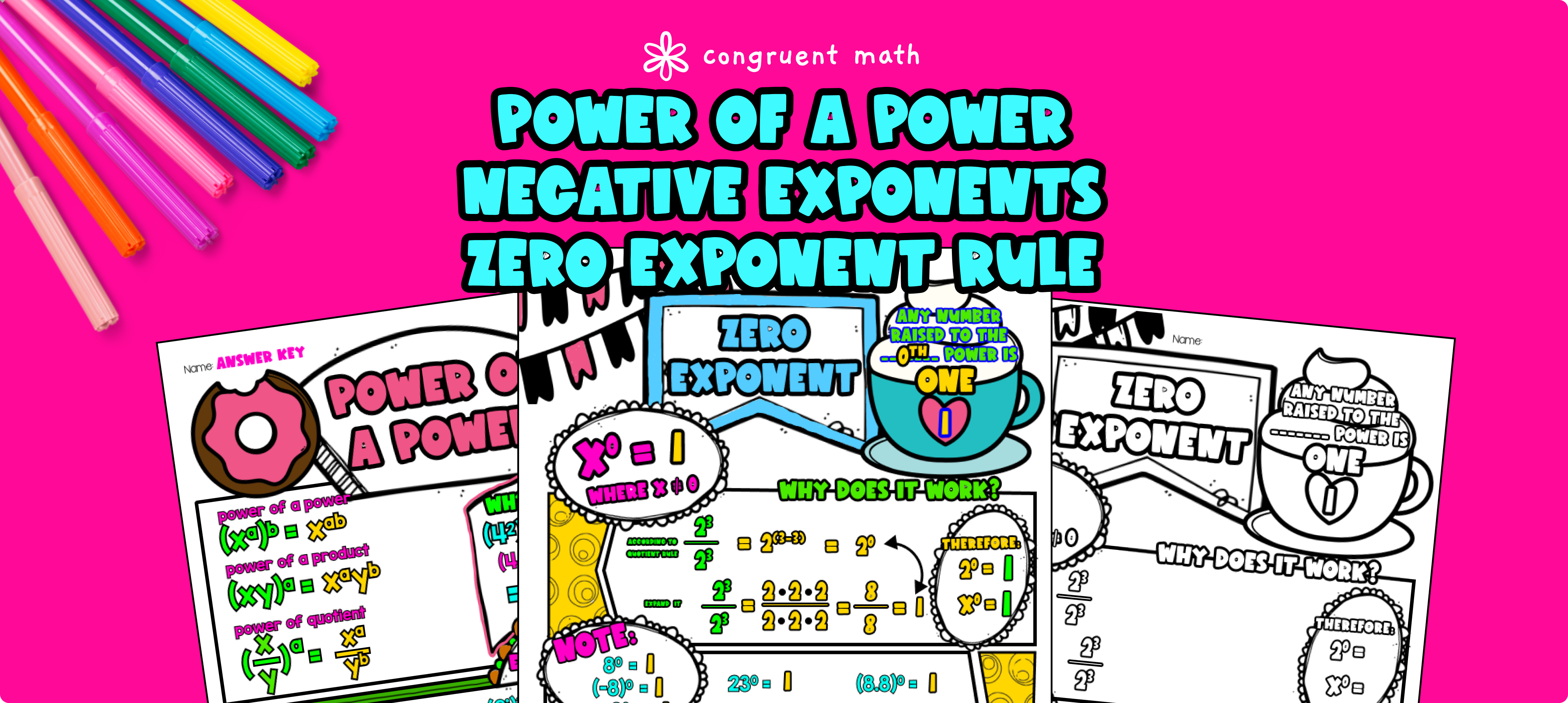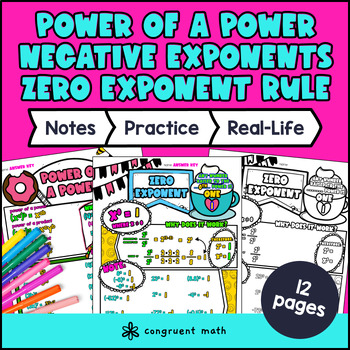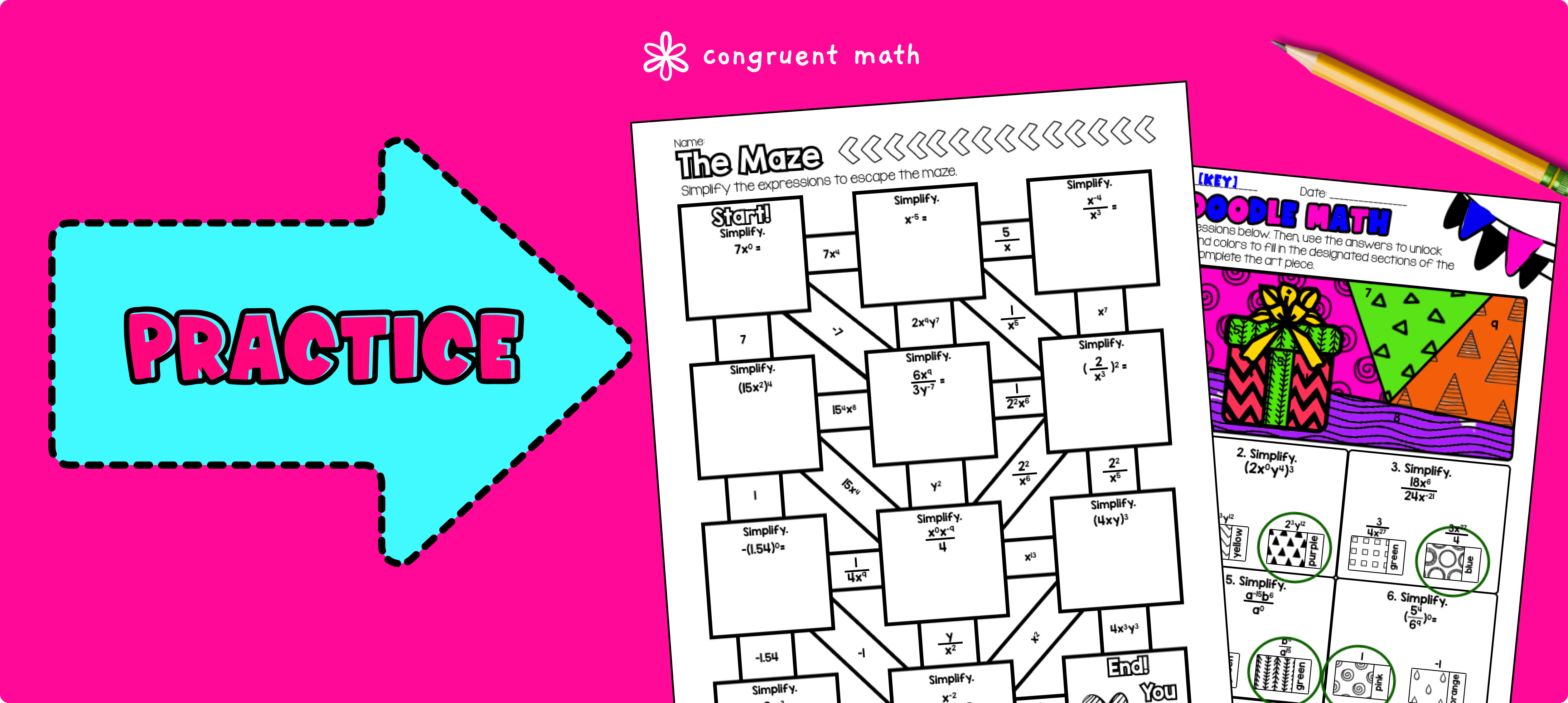# Laws of Exponents: Negative Exponents, Zero Exponents, Power of a Power RulePin This

## Overview

Ever wondered how to teach the Laws of Exponents: Negative Exponents, Zero Exponents, Power of a Power Rule in an engaging way to your 8th grade students? In this lesson plan, students will learn about these concepts and their real-life applications. Through artistic, interactive guided notes, check for understanding, a maze worksheet, and a doodle & color by number activity, students will gain a comprehensive understanding of the Laws of Exponents.

The lesson culminates with a real-life example that explores how compounding interest works in a retirement account.

## Get the Lesson Materials\$4.25

## Learning Objectives

After this lesson, students will be able to:

• Identify the rules for negative exponents, zero exponents, and power of a power in exponential expressions

• Simplify exponential expressions using the laws of exponents

• Apply knowledge of exponential expressions to real-life situations, such as compounding interest in a retirement account

## Prerequisites

Before this lesson, students should be familiar with:

• Basic understanding of parts of an exponential expressions like base and power

• Integer operations

• Understanding of variables and expressions

## Key Vocabulary

• Exponential expression

• Base

• Power

• Negative exponent

• Zero exponent

• Power of a power

## Procedure

### IntroductionPin This

As a hook, ask students why understanding exponential expressions is important. This lesson plan will cover the Laws of Exponents: Negative Exponents, Zero Exponents, and Power of a Power Rule. Students will learn to identify these rules in exponential expressions, simplify expressions using the laws of exponents, and apply their knowledge to real-life situations such as compounding interest in a retirement account.

Walk through each rule using the guided notes and remember to go through a few key examples with your students. Refer to the FAQs below for a walk-through on this, as well as ideas on how to respond to common student questions. There’s a check for understanding integrated into the guided notes. So have students try the practice problems in a group or pairs.

Then, call on students to talk through their answers, potentially on the whiteboard or projector. Based on student responses, reteach concepts that students need extra help with.

### PracticePin This

Have students practice the Laws of Exponents using the Maze activity. Walk around to answer student questions.

Fast finishers can dive into the Doodle & Color by Number activity for extra practice. You can assign it as homework for the remainder of the class.

### Real-Life ApplicationPin This

Bring the class back together, and introduce the concept of compounding interest in a retirement account and how that’s related to exponents. All the information is laid out in the guided notes as well.

Refer to the FAQ for more ideas on how to teach it!

## Extensions

If you’re looking for digital practice for Laws of Exponents, try my Pixel Art activities in Google Sheets. Every answer is automatically checked, and correct answers unlock parts of a mystery picture. It’s incredibly fun, and a powerful tool for differentiation.

Here’s one activities to explore: